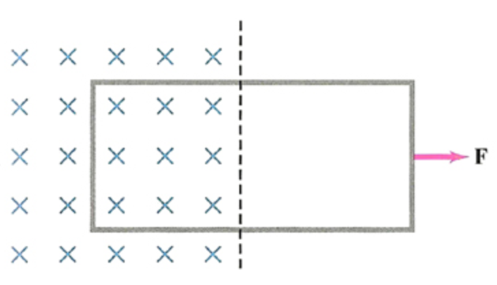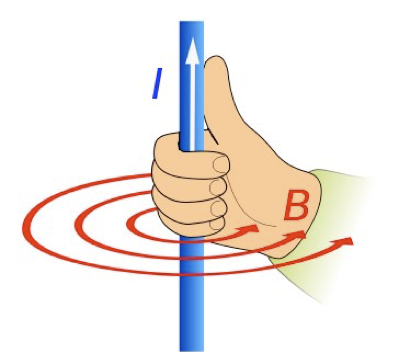# Magnetic Flux and Magnetic Flux Density

This is part of the HSC Physics course under the topic Electromagnetic Induction.

### HSC Physics Syllabus

• describe how magnetic flux can change, with reference to the relationship \phi =B_(||)A=BAcos\theta (ACSPH083, ACSPH107, ACSPH109)

### Magnetic Flux and Flux Density

This video explores what magnetic flux is and how it changes with reference to the relationship \phi =B_(||)A=BAcos\theta.

### What is Magnetic Flux and Flux Density?

Magnetic flux is a measurement of the total number of magnetic field lines passing through a given area. Flux density is a measurement of the density of magnetic field lines. It is another name for the magnetic field strength B. So, Magnetic flux in a given area equals the flux density multiplied by the area.

$$\phi=B_{||}A=BA\cos{\theta}$$

where \theta is the angle between the magnetic field lines and the normal of the surface. From this, we deduce that:

• when the surface is parallel to the magnetic field lines, its normal is perpendicular to the magnetic field (\theta=90°), thus the magnetic flux is zero.
• when the surface is perpendicular to the magnetic field lines, its normal is parallel to the magnetic field (\theta=0°), thus the magnetic flux is maximum.Any changes to the area, magnetic field strength and angle \theta results in a change in magnetic flux passing through the given area.For example, a change in magnetic flux occurs when the area moved to a location with differing magnetic flux, either higher or lower.

 Quantity Magnetic Flux Magnetic Flux Density/Magnetic Field Strength (B) Area (A) Unit(s) Weber (Wb) Tesla (T) or Weber per metre squared (Wbm-2) Metre squared (m2) Scalar or Vector? Vector Vector Scalar

### Currents in Straight Conductors and Solenoids

The direction and orientation of magnetic field accompanying currents in straight conductors and solenoids can be both determined by the right-hand grip rule

 Straight Conductor Solenoid·       Magnetic flux density decreases further away from the straight conductor ·       Constant magnetic flux density in the core of the solenoid. Flux density is reduced outside the solenoid   ·       Direction determined by right-hand grip rule. Thumb points in the direction from south to north pole. Currents flow in the direction of curled fingers.   ·       Effectively used to create electromagnets where the magnitude of the magnetic field B is proportional to the size of the current I

﻿Previous section:﻿ Interaction Between Two Parallel Current-carrying Conductors

Next section: Faraday's Law of Induction